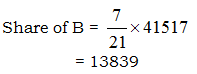Courses

# Quant Mock Test - 12

## 20 Questions MCQ Test Mock Test Series for CLAT 2020 | Quant Mock Test - 12

Description
This mock test of Quant Mock Test - 12 for CLAT helps you for every CLAT entrance exam. This contains 20 Multiple Choice Questions for CLAT Quant Mock Test - 12 (mcq) to study with solutions a complete question bank. The solved questions answers in this Quant Mock Test - 12 quiz give you a good mix of easy questions and tough questions. CLAT students definitely take this Quant Mock Test - 12 exercise for a better result in the exam. You can find other Quant Mock Test - 12 extra questions, long questions & short questions for CLAT on EduRev as well by searching above.
QUESTION: 1

### What value should come in place of question mark (?) in the following number series? 52, 58, 83, 145, 268, 482, ?

Solution: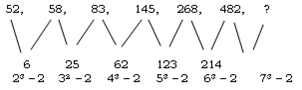So ? = 482 + 73 – 2 ⇒ 823

QUESTION: 2

### If (58)2 is added to the square of a number, the answer thus obtained is 12200. What is the number?

Solution:

(58)2 + ?2 = 12200
?2 = 8836
So ? = 94

QUESTION: 3

### What value should come in place of question mark (?) in the given question?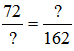Solution:

?2 = 72 x 162
? = 108

QUESTION: 4

In an examination, a candidate must get 65% marks to pass. If a candidate scores 303 marks and is declared failed by 35 marks, then what is the maximum marks?

Solution:

Marks to pass = 303 + 35 = 338
% Marks to pass = 65%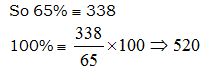QUESTION: 5

The difference between the present age of A and of B is 32 years. If 6 years ago their ages were in the ratio of 3 : 1, then what is the present age of B?

Solution: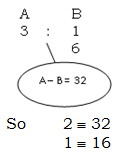So present age of B = 16 + 6 = 22

QUESTION: 6

When 32 is added to 64% of a number, the result is 25% of 576. What is the number?

Solution:

According to question
64% of ? + 32 = 25% of 576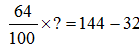So ? = 175

QUESTION: 7

An amount was distributed among A, B and C in the ratio of 7 : 11 : 16. If A received Rs 4986 less than C, then what was the share of B?

Solution:

According to question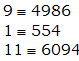QUESTION: 8

A person spent 40% of his monthly salary on house rent and 30% of the remaining amount on food. If the person is left with Rs 3591 then what is his monthly salary?

Solution: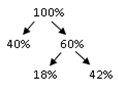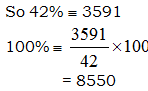QUESTION: 9

In how many different ways can the letters of the word BOTTLE be arranged?

Solution:

Required No. of ways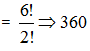QUESTION: 10

What will be the next number in the following number series?

9155, 6961, 5627, 4901, 4555, ?

Solution:

The correct answer is C as
9155 - 6961 = 2194. [2194= 13^3 - 3]
6961 - 5627 = 1334. [1334 = 11^3 +3]
5627 - 4901 = 726 [726 = 9^3 -3]
4901 - 4555 = 692 [7^3 + 3]
4555 - 4433 = 122 [ 5^3 -3]

QUESTION: 11

The average age of 12 persons is 32 years. If the age of one more person is added, the average decreases by one year. What is the age of the new person?

Solution:

Age of New person = (13 x 31) – (32 x 12)
= 19 years

QUESTION: 12

What is the area of a circle whose diameter is 33.6 cm?

Solution:

Area of Circle = πr2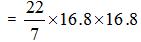= 887.04

QUESTION: 13

A man can row 42 km upstream and 68 km downstream in 10 hours. Also, he can row 56 km upstream and 85 km downstream in 13 hours. What is the speed of the man in still water?

Solution:

Go via options

QUESTION: 14

There is sufficient food for 200 men for 45 days. After 24 days 95 men left. For how many days will the remaining food last for the remaining men?

Solution: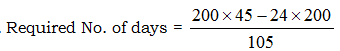= 40 days

QUESTION: 15

Three- sevenths of five-elevenths of four- thirteenths of a number is 960. Then what is the number?

Solution: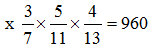? = 16016

QUESTION: 16

A table is sold at a profit of 12%. Had it been sold for Rs 50 more, then there would have been a profit of 20%. What is the cost price of the table?

Solution:

According to question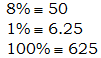QUESTION: 17

A bag contains 7 red, 5 blue and 3 black balls. If two balls are drawn at random, what is the probability that both of them are blue

Solution: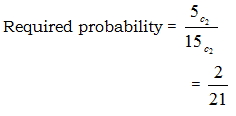QUESTION: 18

When 134 is substracted from a number it is reduced to 7/9 of its value. What is the number?

Solution:

2/9 of Number = 134
So Number = 603

QUESTION: 19

If the length of a rectangle is increased by 24% and its breadth is decreased by 10%, then by what per cent will the area of the rectangle be increased/decreased?

Solution:

Increase/Decrease in Area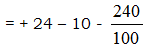= 11.6%

QUESTION: 20

Rs 41517 is to be distributed amongst A, B and C in the ratio of 3 : 7 : 11. What is the share of B?

Solution: